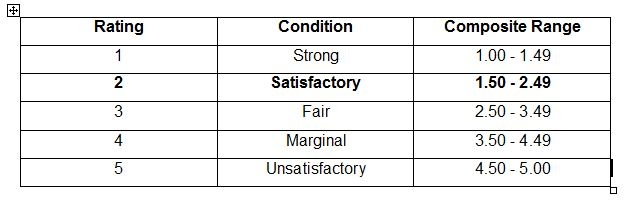# Finance

Retained Earnings:

Retained earnings were placed at Tk. 106.14 million for the year 2004 as against Tk. 44.18 million in the previous year 2003. A year wise graphical prese

ntation of the same is provided on next page:

 Year 2001 2002 2003 2004 Retained Earnings Tk. 8.88 million Tk. 11.63 million Tk. 44.48 million Tk. 106.14 million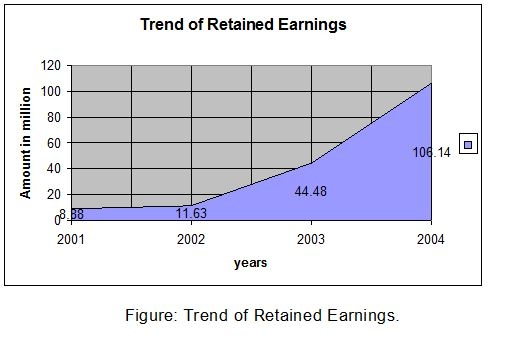Earnings Per share:

Earnings per share in year 2001 was Tk. 17.26 million but decreased in 2002 to Tk. 9.21 million, after that it started to increase and in year 2003 it stood Tk. 17.45 million

In year 2004 it was Tk. 31.71 million.

 Year 2001 2002 2003 2004 EPS Tk. 17.26 million Tk. 9.21 million Tk. 17.45 million Tk. 31.71 million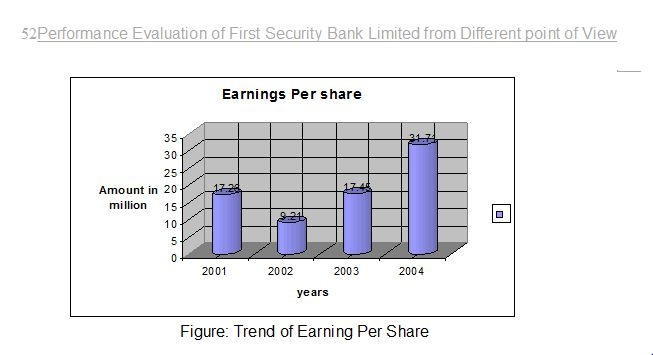Capital funds:

Authorized capital of the bank is tk. 1,000.00 million and paid up capital was tk. 200.00 million in the year 2004. Over the period 2001-2002 the paid –up capital was fixed at tk. 200.00 million and 320 over the period 2003-2004.Reserve Funds: Reserve fund of the bank was tk. 6476.86 million at 2003 and it stood at tk. 8500.00 million as on 31st December 2004 that means it increase at a increasing rate. The reserve fund of First Security Bank Limited for the period 2001-2004 was as follows-Investments: First Security Bank Limiter’s investment portfolio mainly comprised investment in government securities, Treasury bills, Bonds etc.                 (Tk. In million)#### q       Performance Evaluation of First Security Bank Limited from Different Point of View:

From owners viewpoint:

# As an owner of a bank we will analyze three area of a bank:

1.  Profitability of the Bank

2.      Growth of the Bank

3.      Risk of the Bank

1.    Profitability of the Bank:

Operating Profit margin:

This ratio indicates operating income of the bank in relation to total revenue after taking into account operating cost.

Operating Profit margin = Operating profit / Total Revenue.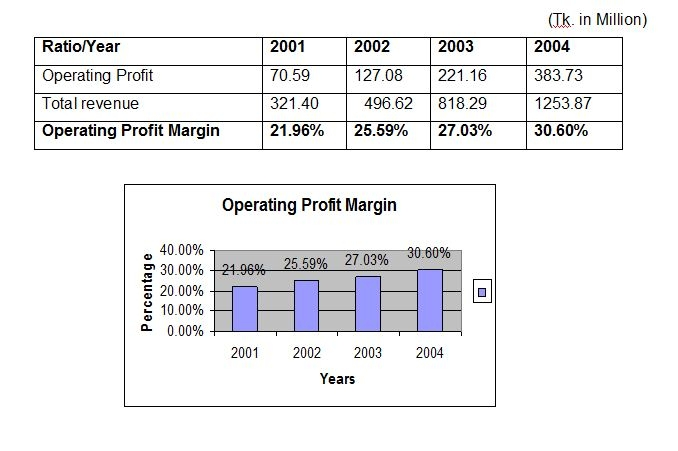Interpretation: In case of First Security Bank Limited we observed that operating profit margin ratio increased gradually over the period this indicates that the bank is operationally efficient.

Net Profit margin: This ratio indicates net income of the bank in relation to total revenue after taking into account operating cost, financial expenses and corporate taxes.               Net Profit margin = Net income / Total Revenue.                 (Tk. in Million)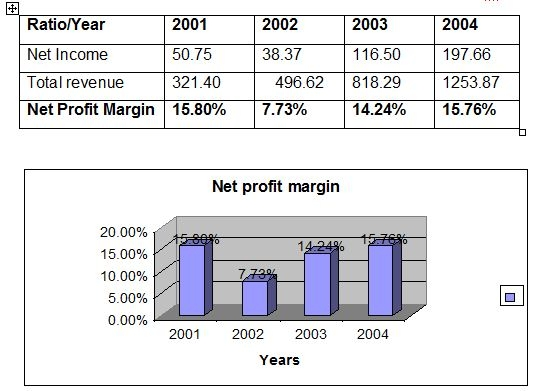Interpretation: In case of First Security Bank Limited, we observed that net profit margin ratio increased over time except year 2002. This ratio has increased due to the decrease of administrative, general expense and income tax relative to revenue.

2. Growth of the Bank:

Return on assets (ROA): Return on assets measure the success of a firm in using assets to generate earning independent of the financing if those assets. This measure therefore separates financing activity from operating and investing activities. RAO is particularly useful in assessing the performance of business segment of firm when as is typical, financing for those segments comes form a central corporate pool of resources.                                          ROA = Net income / Total assets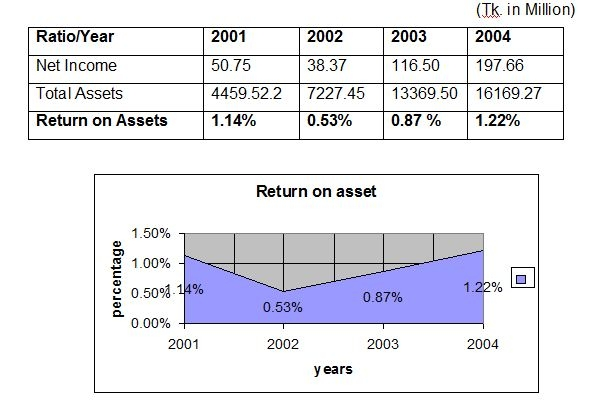Interpretation: The ratio of return on asset of First Security Bank Limited is relatively low; it is lower than 1% over the years 2002 and 2003. It had increased little in 2004 .It indicates that total asset of the bank increased but net profit did not increase with the assets. This shows the management inefficiency in using the bank asset to generate profit.

Return on Equity (ROE): This ratio indicates the degree to which the form is able to convert operating income into an after income that eventually can be claimed by the shareholder. This is a useful ratio for analyzing the ability of the firm’s management to realize an adequate return on the capital invested by the owners of the firm.

ROE = Net income / Total equity                               (Tk. in Million)

Interpretation: From the above data we find that return on equity of FSBL increased in 2004 because the bank has increased its net income as well as the equity.

Provision for loan ratio:

Provision for loan loss / Total operating income                              (Tk. in Million)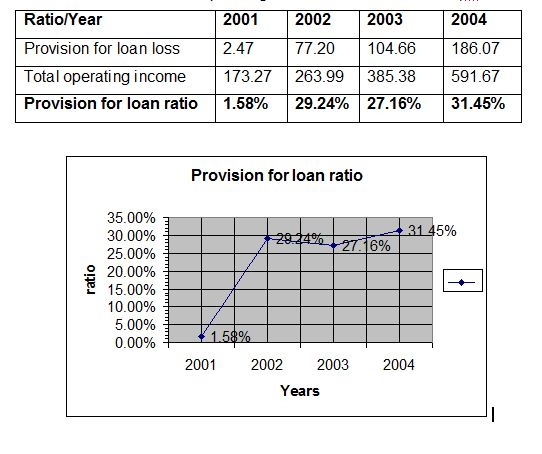Interpretation:

Provision for loan losses ratio of FSBL decreased in 2002, which shows the management efficiency to disburse and recovery of loan.:

# Asset utilization:Asset utilization ratio = Total operating income / Total asset

(Tk. in Million)

 Year 2001 2002 2003 2004 Total operating income 173.27 263.99 385.38 591.67 Total asset 4459.52 7227.45 13369.50 16169.27 Asset utilization ratio 3.89% 3.65% 2.88% 3.66%Interpretation: The data of asset utilization shows that the ratio of FSBL gradually decreased from 2001 to 2003. But in later period it increased in 2004. This indicates that the bank is most inefficient to mobilize profit from total assets.

Interest Income Ratio:

Interest Income Ratio = Interest Income / Total assets            (Tk. in Million)

Ratio/Year 2001 2002 2003 2004

# Interest Income

321.40

496.62

818.29 1253.87
Total assets 4459.52 7227.45 13369.50 16169.27
Interest Income Ratio 7.21% 6.87% 6.12% 7.75%Interpretation: From the above data we see that interest income ratio decreased overtime but little increased in 2004. It indicates that the bank is trying to keep the interest income more stables and they were not successful in this area.

Non-interest Income Ratio: = Non-interest Income / Total assets

### (Tk. in Million)Interpretation: Above table shows the non-interest income of FSBL increased in 2002 because of more activities other than deposit.

Equity Multiplier: Equity multiplier measures the value of assets funded with each taka of equity capital. The higher the ratio, the more leverage or debt the bank is using to fund its assets. Thus the ratio is a measure of the degree of leverage the bank is using.

Equity multiplier = Total assets / Total equity capital

(Tk. in Million)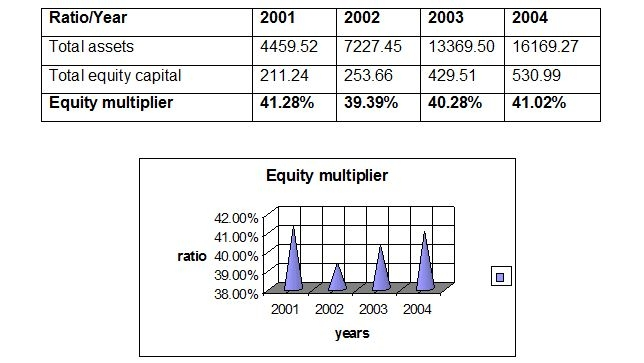Interpretation: Equity multiplier of FSBL was too high, which indicates that the bank was highly depended on leverage.

3. Risk of the Bank:

Interest expense ratio: Interest expense ratio is different method to evaluate the bank’s expense management.

### (Tk. in Million)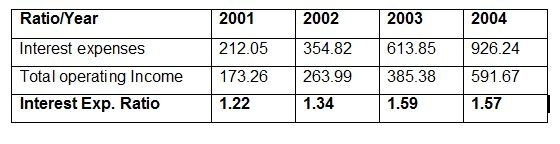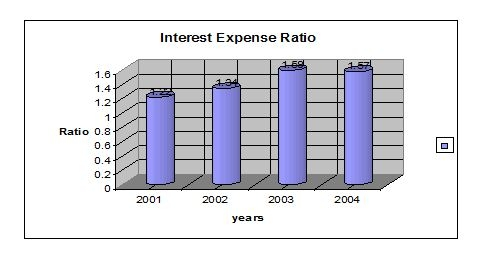Interpretation: From the above data we see that interest expenses ratio was more over stable up to 2002 but increased more in 2003. It indicates that the bank was trying to keep the interest expenses more stables up to 2002but in later period they were unable to maintain the track.

Non-interest expense ratio:

### Non interest expense /Total operating income                                      (Tk. in Million)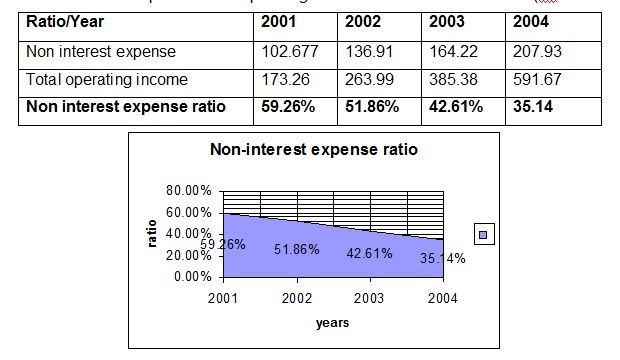Interpretation: Above table shows the non-interest expenses of FSBL was decreasing  over time but it was too high in 2001 because of more activities other than deposit, which had to pay high cost.

Tax ratio:

Tax ratio= Income Taxes / Total operating income

(Tk. in Million)# Interpretation: From 2001 to 2002, the tax ratio decreased, because the total operating income of FSBL was increased.

From the view point of Depositors:

1.            Internal Liquidity

2.Operating Performance

Internal Liquidity: Current Ratio = Current assets / Current liabilities

(Tk. in Million)2. Operating Performance: Capital to deposit ratio = Total Capital / Total deposit

(Tk. in Million)Loan to deposit ratio: Loan to deposit ratio = Total Loan / Total deposit

(Tk. in Million)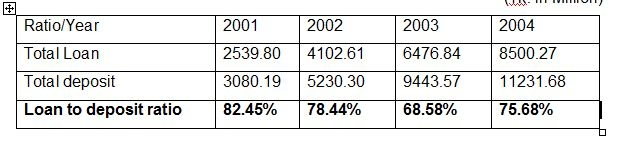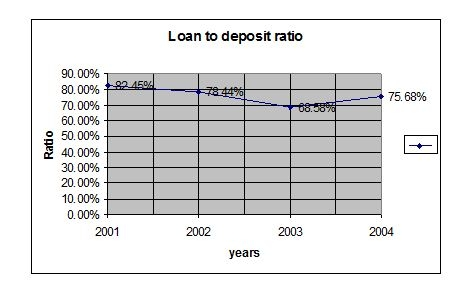Deposit to employee ratio=Total Deposit / Total number of employee

(Tk. in Million)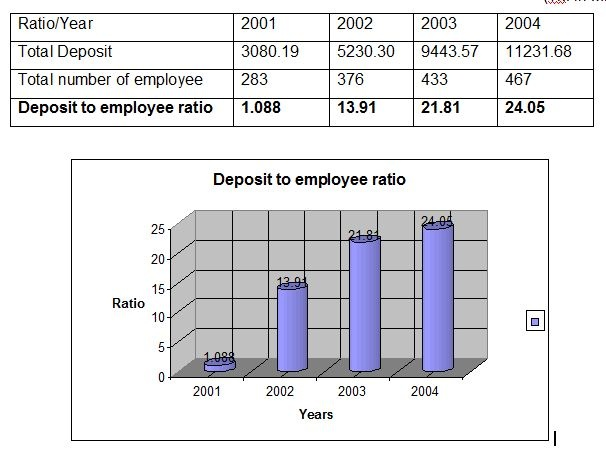CAMEL Rating: CAMEL rating is a suitable way of analyzing bank’s financial performance. It is a technique, which is widely used by the central bank as well as financial analysts to identify bank’s financial position. Five alphabets of CAMEL means:

## A = Asset quality

M = Management efficiency

E = Earnings

L = Liquidity

These cover all major aspects of financial indicators. To appropriately identify bank’s financial position, rating is used which is calculated by following way-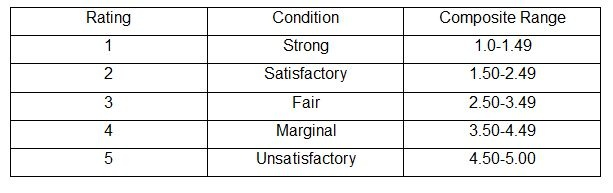Elements of CAMEL rating are calculated step by step in the following:

1. Capital adequacy: Capital adequacy of First Security Bank Limited was 7.57% in year 2004.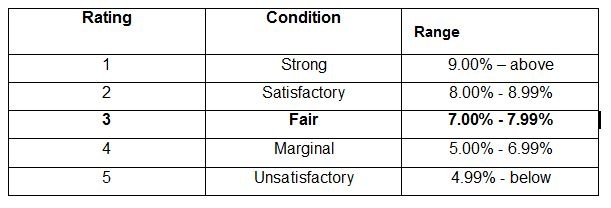Interpretation:

Since the capital adequacy ratio of the First Security Bank Limited in 2004 was 7.57%. Therefore, the capital adequacy of First Security Bank Limited was fair. That means rating was 3.

2. Asset quality: To identify of assets quality of First Security Bank Limited, classification of loans and advance and provision for loans and advances is required to know –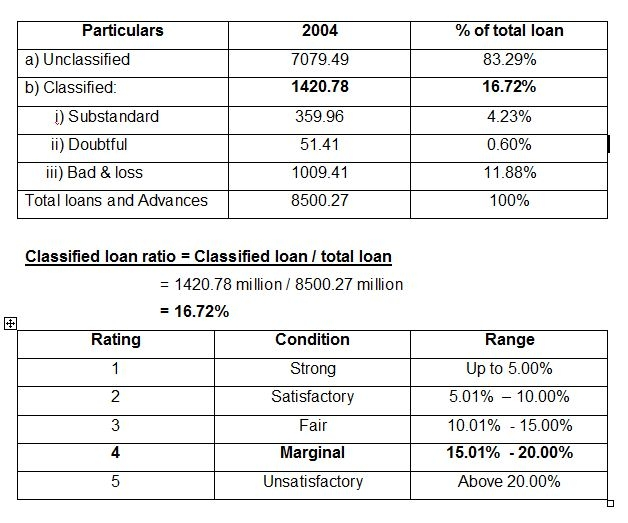Interpretation:

Classified loan ratio of the First Security Bank Limited at 2004 was 16.72%. So, the asset quality of First Security Bank Limited is marginal. That means rating was 4.

Interpretation: Return on assets of the First Security Bank Limited at 2004 was 1.23%. So, the Earnings of First Security Bank Limited were strong. That means rating was 1.

4. Liquidity: Liquidity ratio = total liquid assets / total times demanded liabilities

= 7488.03 million / 60923.1 million = 35.38%Interpretation: Liquidity ratio of the First Security Bank Limited at 2004 was 35.38%. So, the liquidity of First Security Bank Limited was strong. That means rating was 1.

5. Management efficiency:

The management rating is an average of the four ratings given to capital, assets quality, earnings and liquidity.Composite Rating: Composite rating is the average of the all ratings.

Thus, Composite rating = (3+4+2+1+1) / 5   = 2.20